—————  当天上午  —————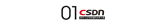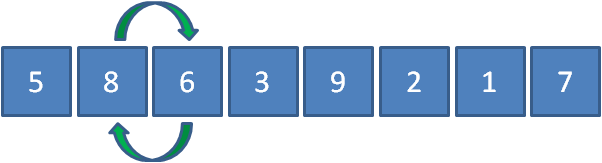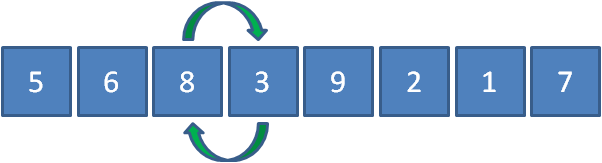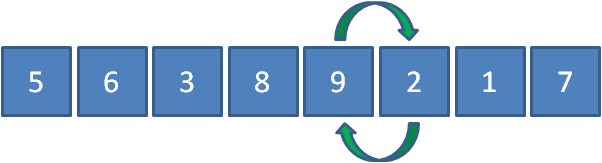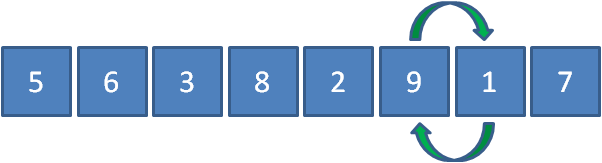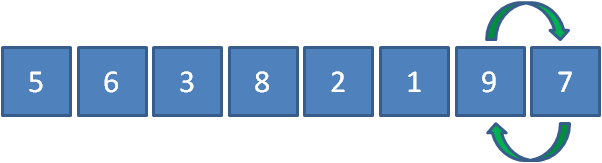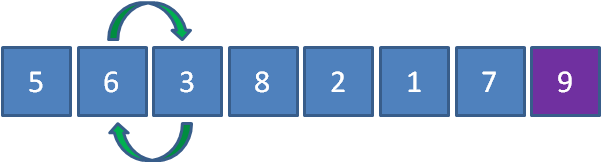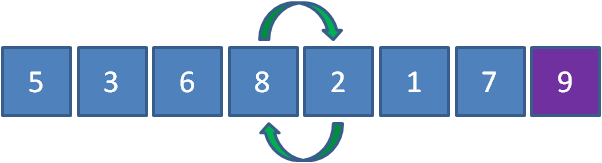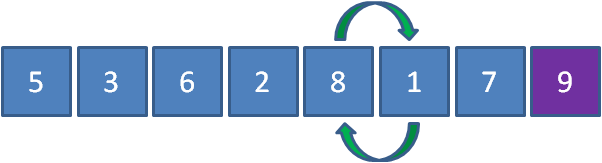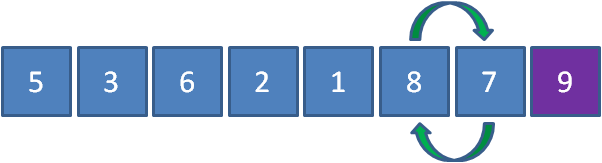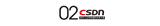1. public class BubbleSort {

2. `private static void sort(int array[])`

3. `{`

4. `    int tmp  = 0;`

5. `        for(int i = 0; i < array.length; i++){`

6. `        for(int j = 0; j < array.length - i - 1; j++)`

7. `        {`

8. `            if(array[j] > array[j+1])`

9. `            {`

10. `                tmp = array[j];`

11. `                array[j] = array[j+1];`

12. `                array[j+1] = tmp;`

13. `            }`

14. `        }`

15. `    }`

16. `}`

17. `public static void main(String[] args){`

18. `    int[] array = new int[]{5,8,6,3,9,2,1,7};`

19. `    sort(array);`

20. `    System.out.println(Arrays.toString(array));`

21. `}`

22. `}`————————————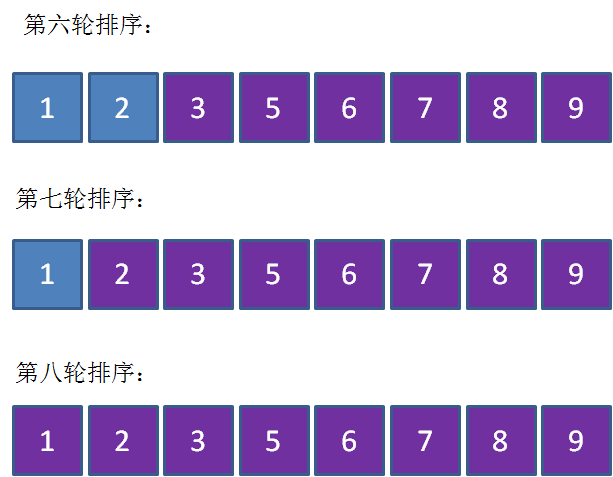1. public class BubbleSort {

2. `private static void sort(int array[])`

3. `{`

4. `    int tmp  = 0;`

5. `    for(int i = 0; i < array.length; i++)`

6. `    {`

7. `        //有序标记，每一轮的初始是true`

8. `        boolean isSorted = true;`

9. `        for(int j = 0; j < array.length - i - 1; j++)`

10. `        {`

11. `            if(array[j] > array[j+1])`

12. `            {`

13. `                tmp = array[j];`

14. `                array[j] = array[j+1];`

15. `                array[j+1] = tmp;`

16. `                //有元素交换，所以不是有序，标记变为false`

17. `                isSorted = false;`

18. `            }`

19. `        }`

20. `        if(isSorted){`

21. `            break;`

22. `        }`

23. `    }`

24. `}`

25. `public static void main(String[] args){`

26. `    int[] array = new int[]{5,8,6,3,9,2,1,7};`

27. `    sort(array);`

28. `    System.out.println(Arrays.toString(array));`

29. `}`

30. `}`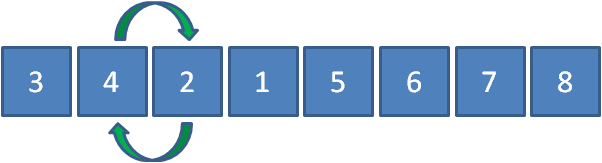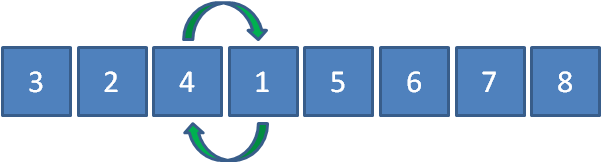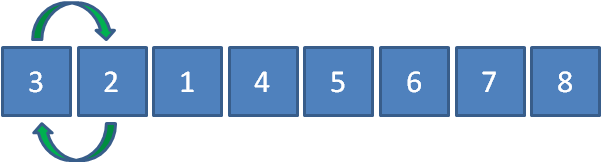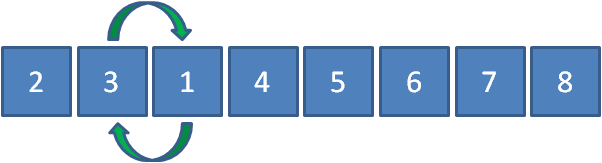1. public class BubbleSort {

2. `private static void sort(int array[])`

3. `{`

4. `    int tmp  = 0;`

5. `    //记录最后一次交换的位置`

6. `    int lastExchangeIndex = 0;`

7. `    //无序数列的边界，每次比较只需要比到这里为止`

8. `    int sortBorder = array.length - 1;`

9. `    for(int i = 0; i < array.length; i++)`

10. `    {`

11. `        //有序标记，每一轮的初始是true`

12. `        boolean isSorted = true;`

13. `        for(int j = 0; j < sortBorder; j++)`

14. `        {`

15. `            if(array[j] > array[j+1])`

16. `            {`

17. `                tmp = array[j];`

18. `                array[j] = array[j+1];`

19. `                array[j+1] = tmp;`

20. `                //有元素交换，所以不是有序，标记变为false`

21. `                isSorted = false;`

22. `                //把无序数列的边界更新为最后一次交换元素的位置`

23. `                lastExchangeIndex = j;`

24. `            }`

25. `        }`

26. `        sortBorder = lastExchangeIndex;`

27. `        if(isSorted){`

28. `            break;`

29. `        }`

30. `    }`

31. `}`

32. `public static void main(String[] args){`

33. `    int[] array = new int[]{3,4,2,1,5,6,7,8};`

34. `    sort(array);`

35. `    System.out.println(Arrays.toString(array));`

36. `}`

37. `}`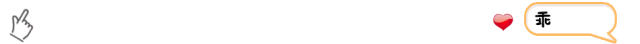20年前，《新程序员》创刊时，我们的心愿是全面关注程序员成长，中国将拥有新一代世界级的程序员。20年后的今天，我们有了新的使命：助力中国IT技术人成长，成就一亿技术人！